## Tuesday, August 31, 2010

### Orthodox Statistics Conducive to Pseudo-Science

I have just realized that the thought process used in orthodox statistics is conducive to pseudo-science. It adds, in my opinion, to the long list of reasons why Bayesian inference is demonstrably superior (also see here). Let me show with a couple of simple examples.

## Astrology

From this skeptical analysis of some astrology data, listing the numbers of famous rich people in each sign, we see the use of the chi-squared goodness of fit test. The data are:

 Sign Number of People Aries 95 Taurus 104 Gemini 110 Cancer 80 Leo 84 Virgo 88 Libra 87 Scorpio 79 Sagittarius 84 Capricorn 92 Aquarius 91 Pisces 73 Total 1067

To apply the chi-squared test, we simply compare the above numbers to the expected numbers if completely random, which is 1067 people/12=88.9 people according to: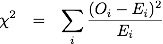where O are the observed data and E are the expected counts. Once we have the chi-square value and the degrees of freedom (11 in this case), we can look up in tables to get the p-value: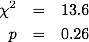Normally, this might be the end of the story, given that there is not even close to a significant value (usual cut-off around p=0.05).

### Subset of the Data

So, if we only take the extreme values, say:

 Sign Number of People Gemini 110 Pisces 73 Total 183

then we calculate a different chi-squared, with 1 degree of freedom, and get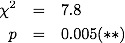Now this is pretty silly: of course, if you take the extreme values of 12 numbers, and pretend that they came from a 2-category situation, then it'll appear more significant. What about lumping 6 points together, say Capricorn to Gemini (the first part of the year) and the second part. In this case we aren't cherry picking, and the sums should be less significant than the individual data. We then have:

 Sign Number of People Capricorn-Gemini 565 Cancer-Sagittarius 502 Total 1067

And we expect 533.5 people in each category. Notice that we went from (the most extreme) 20 person difference from expected in about 100 to a 30 person difference in 500...closer to the expected. What do we get from our chi-squared test?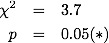The test says that this is significantly different from random, more than the individual data! At least the goodness of fit measure, chi-squared value, went down to denote a closer fit to expected but the reduction in the number of data points changes the test quite a lot.

### A different measure

E.T. Jaynes suggests in his book to use a different measure of goodness of fit, the &psi measure closely related to the log-likelihood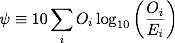Using this measure on the above examples, we get

• All data: &psi = 28.9
• Extreme data: &psi = 39.1
• Lumped data: &psi = 8.1
which is completely in agreement with our intuition. The chi-squared test does not match our intuition, and seems to give significance to things that we know shouldn't be. But what about the test with the &psi-measure? How can we tell whether it is a significant difference? One could, in theory, give an arbitrary threshold but that would not be particularly useful, and would not be what a Bayesian would do. What a Bayesian would do is compare values of the goodness-of-fit measure to different models on the same data. It makes no sense, if you have only one model, to reject it by a statistical test...reject it in favor of what? If you have only one model, say Newton's Laws, and you have data that are extremely unlikely given that model, say the odd orbit of Mercury, you don't simply reject Newton's Laws until you have something else to put on the table. The either-or thinking of orthodox statistical tests is very similar to the either-or thinking of the pseudoscientist: either it is random, or it is due to some spiritual, metaphysical, astrological effect. You reject random, and thus you are forced to accept the only alternative put forward. I am not implying that all statisticians are supportive of pseudo-science, and they are often the first to say that you can only reject hypotheses not confirm them. However, since the method of using statistical tests does not stress the searching for alternatives, or better, the necessity for alternatives, it is conducive to these kinds of either-or logical fallacies. An example of a model comparison, from a Bayesian perspective, on a problem suffering from either-or fallacies can be found in the non-psychic octopus post I did earlier.

#### 1 comment:

1.An elementary question about the motivation for the chi-square formula:

If I were to create something like the chi-squared statistic from scratch, I'd base it on the square of the "z-score" of the observed data in a bin. If the there are N observations and each has a probability of p of landing in the bin then the z-score when O observations are in the bin would be (O - Np)/ sqrt ( Np(1-p)) and the square of that would be (O - E)^2/ (E(1-p)) where E = Np is the expected number of observations in the bin. So there would be a factor of (1-p) in the denominator that is missing from the chi-squared statistic. is my algebra wrong? - or Is there an intuitive explanation of why the factor of (1-p) is left out?